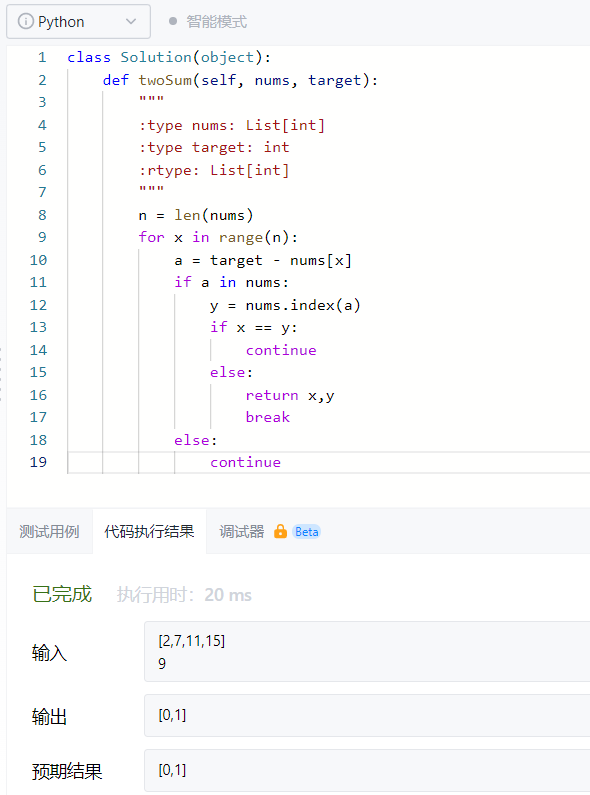###### 賈努力
2021-12-19 23:49``````class Solution:
def twoSum(self,nums, target):
"""
:type nums: List[int]
:type target: int
:rtype: List[int]
"""

n = len(nums)                  #len()取得nums列表长度
for x in range(n):             #x从0到n取值（不包括n）
a = target - nums[x]
if a in nums:            #用in关键字查询nums列表中是否有a
y = nums.index(a)      #用index函数取得a的值在nums列表中的索引
if x == y:             #假如x=y,那么就跳过,否则返回x,y
continue
else:
return x,y
break
else :
continue``````

知识点：

1.break语句用来终止循环语句，即循环条件没有False条件或者序列还没被完全递归完，也会停止执行循环语句。
continue 语句用来告诉Python跳过当前循环的剩余语句，然后继续进行下一轮循环。

2.index() 函数用于从列表中找出某个值第一个匹配项的索引位置

index()方法语法：list.index(x[, start[, end]])

x-- 查找的对象。
start-- 可选，查找的起始位置。
end-- 可选，查找的结束位置。

• 写回答
• 好问题 提建议
• 追加酬金
• 关注问题
• 邀请回答

#### 1条回答默认 最新

•Whimsy长律 2021-12-30 20:29
最佳回答

哈希表做 跑的贼快

``````class Solution(object):
def twoSum(self, nums, target):
"""
:type nums: List[int]
:type target: int
:rtype: List[int]
"""
nums_hash = {}
nums_len = len(nums)
for i in range(nums_len):
dif = target - nums[i]
if dif in nums_hash:
return [nums_hash[dif], i]
nums_hash[nums[i]] = i
return []

``````
评论
解决 1 无用
打赏 举报